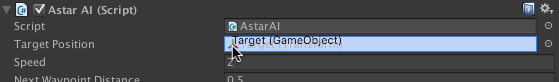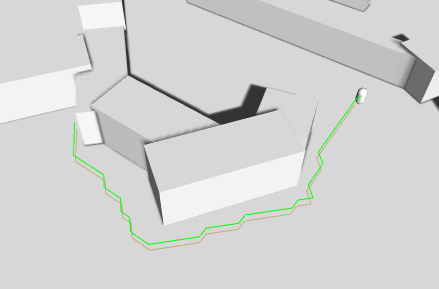# Writing a movement script

Tutorial for writing a simple custom movement script.

This tutorial is part of the get started tutorial: Get Started With The A* Pathfinding Project.

We are going to write our own, really simple script for moving the AI, so open your favourite script-editor and follow.

### Note

This tutorial can be followed both if you are creating a 3D game as well as a 2D game. In some cases there are slightly different instructions for 2D and 3D. The code for 2D and 3D are almost the same, but there are a few places in which they differ. These cases are clearly indicated using comments. The environment created in the get started tutorial is however a 3D environment. For instructions on how to create a graph for a 2D environment take a look at Pathfinding in 2D.

The first thing we need to do is to calculate a path. For this we use the StartPath method on the Seeker component. The call to the Seeker is really simple, three arguments, a start position, an end position and a callback function (must be in the form "void SomeFunction (Path p)"): `Path StartPath (Vector3 start, Vector3 end, OnPathDelegate callback = null) `

So let's start our script with a simple snippet that starts a path request at startup: `using UnityEngine;using System.Collections;// Note this line, if it is left out, the script won't know that the class 'Path' exists and it will throw compiler errors// This line should always be present at the top of scripts which use pathfindingusing Pathfinding;public class AstarAI : MonoBehaviour { public Transform targetPosition; public void Start () { // Get a reference to the Seeker component we added earlier Seeker seeker = GetComponent<Seeker>(); // Start to calculate a new path to the targetPosition object, return the result to the OnPathComplete method. // Path requests are asynchronous, so when the OnPathComplete method is called depends on how long it // takes to calculate the path. Usually it is called the next frame. seeker.StartPath(transform.position, targetPosition.position, OnPathComplete); } public void OnPathComplete (Path p) { Debug.Log("Yay, we got a path back. Did it have an error? " + p.error); }} `

Save it to a file in your project named AstarAI.cs and add the script to the AI GameObject.
Create a new GameObject that we can use as a target, move it to the coordinates say (-20,0,22) and then select the AI GameObject and drag the target to the targetPosition field. This is the position the AI will try to find a path to now.Press Play. You should get the log message and also the path should show up in the scene view as a green line (the Seeker component draws the last calculated path using Gizmos).

If you do not see a green line, make sure that the checkbox Show Gizmos on the Seeker component is checked. More recent Unity versions also depth-test gizmos, so it might be hidden under the ground, to disable the depth-testing click the Gizmos button above the scene view window and uncheck the '3D Icons' checkbox.In case you get an error, make sure that the Seeker component really is attached to the same GameObject as the AstarAI script. If you still get an error, the target position might not be reachable, try to change it a bit.

### SeeIt doesn't look very smooth, but that will do for now as you might be waiting for an explanation of what that code really did.

What happens is that first the script calls the Seeker's StartPath method. The seeker will then create a new ABPath instance and then send it to the AstarPath script (the Pathfinder component you added before). The AstarPath script will put the path in a queue. As soon as possible, the script will then process the path by searching the grid, node by node until the end node is found.

Once calculated, the path is returned to the Seeker which will post process it if any modifiers are attached and then the Seeker will call the callback function specified in the call. The callback is also sent to Seeker.pathCallback which you can register to if you don't want to specify a callback every time you call StartPath: `// OnPathComplete will be called every time a path is returned to this seekerseeker.pathCallback += OnPathComplete;//So now we can omit the callback parameterseeker.StartPath(transform.position, targetPosition);`

### Note

When disabling or destroying the script, callback references are not removed, so it is good practise to remove the callback during OnDisable in case that should happen
`public void OnDisable () { seeker.pathCallback -= OnPathComplete;}`

When we get the calculatated path back, how can we get info from it?

A Path instance contains two lists related to that. Path.vectorPath is a Vector3 list which holds the path, this list will be modified if any smoothing is used, it is the recommended way to get a path. secondly there is the Path.path list which is a list of GraphNode elements, it holds all the nodes the path visisted which can be useful to get additonal info on the traversed path.

First though, you should always check path.error, if that is true, the path has failed for some reason. The field Path.errorLog will have more info about what went wrong in case path.error is true.

To expand our AI script, let's add some movement.

3D2D
We will move the agent using the Unity built-in component CharacterController. So attach a CharacterController to the AI GameObject.We will move the agent by simply modifying the position of the Transform component.

The script will keep track of the waypoint in the path that it is moving towards and then change that to the next one as soon as it gets close to one.

Every frame we will do a few things:

• First we check if we have a calculated path to follow. Since path requests are asynchronous it may take a few frames (usually one) for the path to be calculated.

• Then we check if the agent is close to the waypoint that it is currently moving towards, and if it is we switch to the next waypoint and repeat the check.

• To calculate how to move we take the coordinate of the current waypoint and subtract our position from that. This will give us a vector pointing towards the waypoint. We normalize that vector to make it have a length of 1, otherwise we would move faster the further away from the waypoint we were.

• We then multiply that vector with our speed to get a velocity.

• Finally we move the agent by using the CharacterController.SimpleMove method (or by modifying transform.position if you are creating a 2D game).

3D2D
The script below works for 3D games To make the script below work for 2D games: look in the comments for 'If you are writing a 2D game' and make the necessary changes.

`using UnityEngine;// Note this line, if it is left out, the script won't know that the class 'Path' exists and it will throw compiler errors// This line should always be present at the top of scripts which use pathfindingusing Pathfinding;[HelpURL("http://arongranberg.com/astar/documentation/beta/class_partial1_1_1_astar_a_i.php")]public class AstarAI : MonoBehaviour { public Transform targetPosition; private Seeker seeker; private CharacterController controller; public Path path; public float speed = 2; public float nextWaypointDistance = 3; private int currentWaypoint = 0; public bool reachedEndOfPath; public void Start () { seeker = GetComponent<Seeker>(); // If you are writing a 2D game you should remove this line // and use the alternative way to move sugggested further below. controller = GetComponent<CharacterController>(); // Start a new path to the targetPosition, call the the OnPathComplete function // when the path has been calculated (which may take a few frames depending on the complexity) seeker.StartPath(transform.position, targetPosition.position, OnPathComplete); } public void OnPathComplete (Path p) { Debug.Log("A path was calculated. Did it fail with an error? " + p.error); if (!p.error) { path = p; // Reset the waypoint counter so that we start to move towards the first point in the path currentWaypoint = 0; } } public void Update () { if (path == null) { // We have no path to follow yet, so don't do anything return; } // Check in a loop if we are close enough to the current waypoint to switch to the next one. // We do this in a loop because many waypoints might be close to each other and we may reach // several of them in the same frame. reachedEndOfPath = false; // The distance to the next waypoint in the path float distanceToWaypoint; while (true) { // If you want maximum performance you can check the squared distance instead to get rid of a // square root calculation. But that is outside the scope of this tutorial. distanceToWaypoint = Vector3.Distance(transform.position, path.vectorPath[currentWaypoint]); if (distanceToWaypoint < nextWaypointDistance) { // Check if there is another waypoint or if we have reached the end of the path if (currentWaypoint + 1 < path.vectorPath.Count) { currentWaypoint++; } else { // Set a status variable to indicate that the agent has reached the end of the path. // You can use this to trigger some special code if your game requires that. reachedEndOfPath = true; break; } } else { break; } } // Slow down smoothly upon approaching the end of the path // This value will smoothly go from 1 to 0 as the agent approaches the last waypoint in the path. var speedFactor = reachedEndOfPath ? Mathf.Sqrt(distanceToWaypoint/nextWaypointDistance) : 1f; // Direction to the next waypoint // Normalize it so that it has a length of 1 world unit Vector3 dir = (path.vectorPath[currentWaypoint] - transform.position).normalized; // Multiply the direction by our desired speed to get a velocity Vector3 velocity = dir * speed * speedFactor; // Move the agent using the CharacterController component // Note that SimpleMove takes a velocity in meters/second, so we should not multiply by Time.deltaTime controller.SimpleMove(velocity); // If you are writing a 2D game you should remove the CharacterController code above and instead move the transform directly by uncommenting the next line // transform.position += velocity * Time.deltaTime; }}` If you press play now, the AI will follow the calculated path, neat, eh?

If you want you can attach the SimpleSmoothModifier to the GameObject to get a smoother path. You can read more about modifiers in Using Modifiers.

Here is a video of the movement script in action in a 2D scene with a simple smooth modifier to smooth out the path:

You can find a few additional improvements such as recalculating the path regularly here: AstarAI.cs.

This is the end of this tutorial. I recommend that you continue with the rest of the get started tutorial: Back to the get started tutorial.